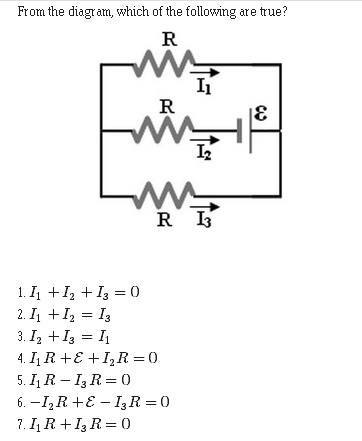# [Highschool Physics] Kirchoff's Rules

## Homework Statement

http://imgur.com/XXPWbCN

## Homework Equations

∑ ΔV (closed circuit) = 0
∑ I (in) = ∑ I (out)

## The Attempt at a Solution

So I know that the sum of the currents entering any junction in a circuit must equal the sum of the currents leaving that junction, and that the sum of the potential differences across all elements around any closed circuit loop must be zero. BUT this picture is so weird that I can't wrap my head around it. I think that I1 + I2 + I3 = 0, if one of those was negative which it can be. This means that (2) and (3) cannot be true. Then, solving for the potential difference equations, I got epsilon=-RI1+RI2 = -RI3+RI2, so I1R-I3R=0. So my answer would be both (1) and (5), but I'm not sure if that is correct. Could anyone help?

So I know that the sum of the currents entering any junction in a circuit must equal the sum of the currents leaving that junction, and that the sum of the potential differences across all elements around any closed circuit loop must be zero. BUT this picture is so weird that I can't wrap my head around it. I think that I1 + I2 + I3 = 0, if one of those was negative which it can be. This means that (2) and (3) cannot be true. Then, solving for the potential difference equations, I got epsilon=-RI1+RI2 = -RI3+RI2, so I1R-I3R=0. So my answer would be both (1) and (5), but I'm not sure if that is correct. Could anyone help?

Correct .

SammyS
Staff Emeritus
Homework Helper
Gold Member

## Homework Statement

http://imgur.com/XXPWbCN

## Homework Equations

∑ ΔV (closed circuit) = 0
∑ I (in) = ∑ I (out)

## The Attempt at a Solution

So I know that the sum of the currents entering any junction in a circuit must equal the sum of the currents leaving that junction, and that the sum of the potential differences across all elements around any closed circuit loop must be zero. BUT this picture is so weird that I can't wrap my head around it. I think that I1 + I2 + I3 = 0, if one of those was negative which it can be. This means that (2) and (3) cannot be true. Then, solving for the potential difference equations, I got epsilon=-RI1+RI2 = -RI3+RI2, so I1R-I3R=0. So my answer would be both (1) and (5), but I'm not sure if that is correct. Could anyone help?
Due to my OCD, I like the images to be visible -- or at least documented.